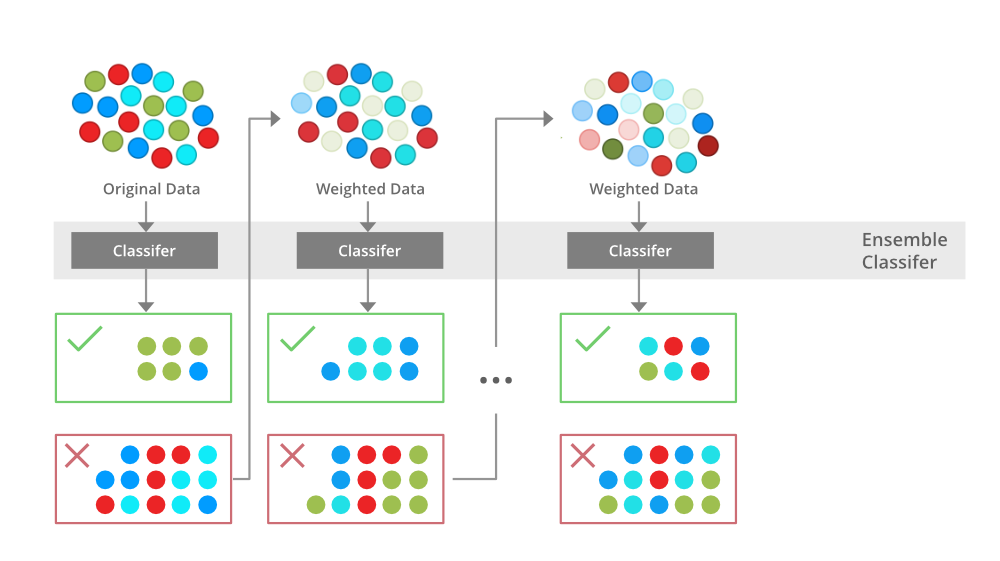GFG App
Open AppBrowser
Continue

# Boosting in Machine Learning | Boosting and AdaBoost

A single weak model may not be enough for our complex problems such cases we aggregate various weak models to make a powerful and more accurate model for our problem this process of aggregating several small problems to create a strong model is what we do in boosting.

## What is Boosting

Boosting is an ensemble modeling technique that attempts to build a strong classifier from the number of weak classifiers. It is done by building a model by using weak models in series. Firstly, a model is built from the training data. Then the second model is built which tries to correct the errors present in the first model. This procedure is continued and models are added until either the complete training data set is predicted correctly or the maximum number of models are added.

• Improved Accuracy – Boosting can improve the accuracy of the model by combining several weak models’ accuracies and averaging them for regression or voting over them for classification to increase the accuracy of the final model.
• Robustness to Overfitting – Boosting can reduce the risk of overfitting by reweighting the inputs that are classified wrongly.
• Better handling of imbalanced data – Boosting can handle the imbalance data by focusing more on the data points that are misclassified
• Better Interpretability – Boosting can increase the interpretability of the model by breaking the model decision process into multiple processes.

### Training of Boosting Model

1. Initialise the dataset and assign equal weight to each of the data point.
2. Provide this as input to the model and identify the wrongly classified data points.
3. Increase the weight of the wrongly classified data points.
4. if (got required results)
Goto step 5
else
Goto step 2
5. EndTraining a boosting model

The Explanation for Training the Boosting Model:
The above diagram explains the AdaBoost algorithm in a very simple way. Let’s try to understand it in a stepwise process:

• B1 consists of 10 data points which consist of two types namely plus(+) and minus(-) and 5 of which are plus(+) and the other 5 are minus(-) and each one has been assigned equal weight initially. The first model tries to classify the data points and generates a vertical separator line but it wrongly classifies 3 plus(+) as minus(-).
• B2 consists of the 10 data points from the previous model in which the 3 wrongly classified plus(+) are weighted more so that the current model tries more to classify these pluses(+) correctly. This model generates a vertical separator line that correctly classifies the previously wrongly classified pluses(+) but in this attempt, it wrongly classifies three minuses(-).
• B3 consists of the 10 data points from the previous model in which the 3 wrongly classified minus(-) are weighted more so that the current model tries more to classify these minuses(-) correctly. This model generates a horizontal separator line that correctly classifies the previously wrongly classified minuses(-).
• B4 combines together B1, B2, and B3 in order to build a strong prediction model which is much better than any individual model used.

### Types Of Boosting Algorithms

There are several types of boosting algorithms some of the most famous and useful models are as :

1. Gradient Boosting – It is a boosting technique that builds a final model from the sum of several weak learning algorithms that were trained on the same dataset. It operates on the idea of stagewise addition. The first weak learner in the gradient boosting algorithm will not be trained on the dataset; instead, it will simply return the mean of the relevant column. The residual for the first weak learner algorithm’s output will then be calculated and used as the output column or target column for the next weak learning algorithm that will be trained. The second weak learner will be trained using the same methodology, and the residuals will be computed and utilized as an output column once more for the third weak learner, and so on until we achieve zero residuals. The dataset for gradient boosting must be in the form of numerical or categorical data, and the loss function used to generate the residuals must be differential at all times.
2. XGBoost – In addition to the gradient boosting technique, XGBoost is another boosting machine learning approach. The full name of the XGBoost algorithm is the eXtreme Gradient Boosting algorithm, which is an extreme variation of the previous gradient boosting technique. The key distinction between XGBoost and GradientBoosting is that XGBoost applies a regularisation approach. It is a regularised version of the current gradient-boosting technique. Because of this, XGBoost outperforms a standard gradient boosting method, which explains why it is also faster than that. Additionally, it works better when the dataset contains both numerical and categorical variables.
3. Adaboost – AdaBoost is a boosting algorithm that also works on the principle of the stagewise addition method where multiple weak learners are used for getting strong learners. The value of the alpha parameter, in this case, will be indirectly proportional to the error of the weak learner, Unlike Gradient Boosting in XGBoost, the alpha parameter calculated is related to the errors of the weak learner, here the value of the alpha parameter will be indirectly proportional to the error of the weak learner.
4. CatBoost – The growth of decision trees inside CatBoost is the primary distinction that sets it apart from and improves upon competitors. The decision trees that are created in CatBoost are symmetric. As there is a unique sort of approach for handling categorical datasets, CatBoost works very well on categorical datasets compared to any other algorithm in the field of machine learning. The categorical features in CatBoost are encoded based on the output columns. As a result, the output column’s weight will be taken into account while training or encoding the categorical features, increasing its accuracy on categorical datasets.